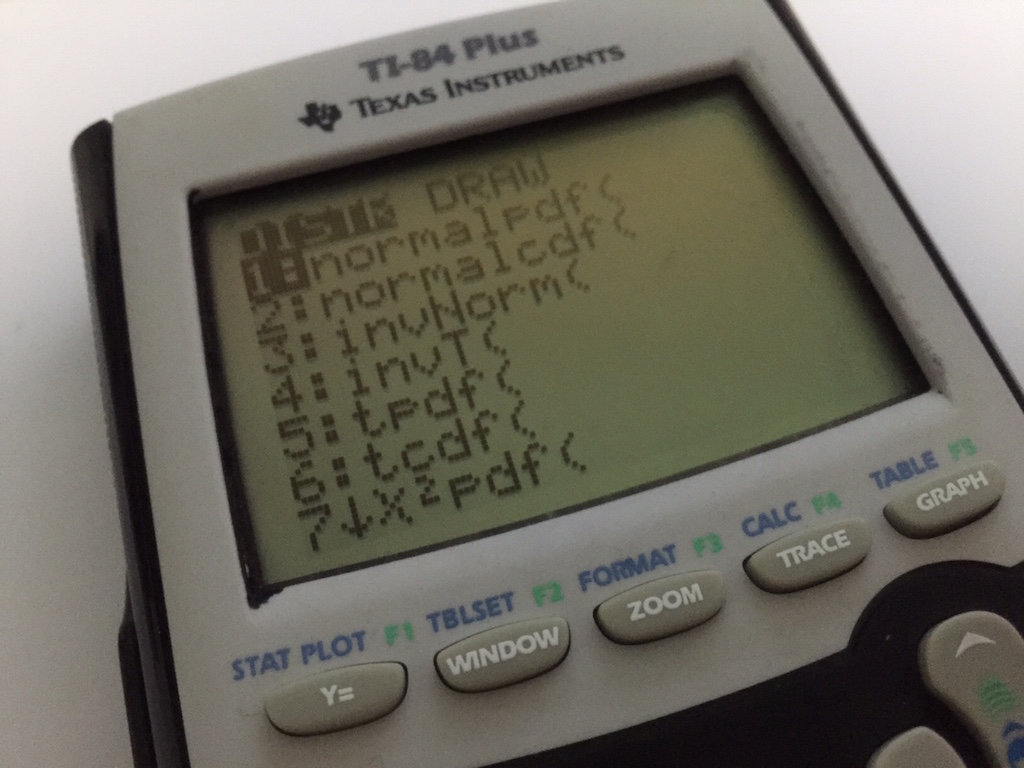# MF15 can’t be more useful!The only cheatsheet every student can bring into the exam hall.

### What is MF15?

It’s the formula sheet every H2 Math student needs to know about.

View PDF

I thought we can look at the MF15 and also highlight a few common mistakes that students make in exams. 🙂

Firstly, the trigonometry formulas are all given in exams. Most students however, do not know what they have. I strongly suggest students spend time to know what they have. And if you’re interested, you should really learn how to derive the sum to product formula from the product to sum formula. You can read more here.

Secondly, the integration formulas that students see in MF15, are not as innocent as they look. Many students overlook the fact that the coefficient of theis ONE! You can read up more here. too.

Lastly, the r-formula is given. Students should know how to use it at the least. Also the properties of the Binomial and Poisson distribution are given (if you’re J2 and still need to refer to know the E(X) and Var(X), you should be worried too).And if you’re not using TI-84, I strongly suggest you learn how to read the T-Table and Z-Table.

Not readable? Change text.